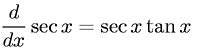Equations > Calculus > Differentiation/Differentals > Derivative of Secant

### Derivative of SecantLatex Code:

MathML Code:

 $\fracd\mathrm{dx}\mathrm{sec}x=\mathrm{sec}x\mathrm{tan}x$

MathType 5.0: